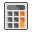Expert Modelling: Hands-on help from Rocscience Engineers. Register Here

# Compute

To compute results for your RS2 model, select the Computeoption from the toolbar or the Analysis menu. This will carry out the finite element stress analysis for the current model. NOTE:

• The Compute option is not enabled unless the finite element mesh exists.
• If you select Compute before saving a file, you will first be prompted to save the file, and then the analysis will be run.
• If for some reason you need to abort the analysis after selecting Compute, select the Abort button on the Compute dialog, and the analysis will be terminated.
• If you are modeling a finite element seepage analysis, the seepage analysis is automatically computed when you select Compute. You can also compute groundwater independently of the stress analysis by selecting the Compute Groundwater option.
• The RS2 analysis engine can also be run as a standalone program (e.g. for batch file compute) as described below.

## Compute Engine

Two different compute engines (parallel and sequential) are provided with the RS2 program:

1. The default engine utilizes multi-core parallel processing techniques. Depending on the number of CPU cores in your computer, this approach can lead to considerable speed gains. Filename: feawin.exe
2. A sequential processing engine (non-parallel, single core) which forces the engine to use sequential rather than parallel processing. Filename: feawin_seq.exe

The executable files can be found in your RS2 installation folder.

When you select the Computeoption in the RS2 model program, the compute engine which is launched is determined by the setting of the Use multi-core processing for compute engine check box in the Preferences dialog:

• If this check box is turned ON, the multi-core parallel processing engine will be used (feawin.exe).
• If this check box is turned OFF, the sequential processing engine will be used (feawin_seq.exe).

The multi-core parallel processing engine is the default compute engine, with significantly faster solution times for large models. In general the multi-core parallel engine is recommended. If you wish to use the sequential compute engine, you can turn off this check box in Preferences.

NOTE:

• One consequence of multi-core parallel processing is that analysis results may vary slightly, if you re-compute the analysis. This is normal, and differences between runs should be insignificant.
• If you use the sequential compute engine, you should always obtain exactly the same analysis results if you re-compute.
• The speed advantage of using the parallel processing engine becomes more significant as the size and complexity of your RS2 model increases.
• If you are performing SSR stability analysis with large complex models, the parallel processing engine will provide the maximum benefit, and can significantly decrease overall computation time required for analysis.

Running Compute as a Standalone Program

The executable files for the compute engines are found in your RS2 installation folder, and have the following filenames:

• feawin.exe (multi-core parallel processing compute engine)
• feawin_seq.exe (single core sequential compute engine)

You can run either engine as a standalone program (e.g. for batch file compute) by double-clicking on the desired filename.

This will display the Compute dialog, allowing you to select one or more files to compute.

## Output Files

After a RS2 analysis, the following output files are created:

 .R?? The .R?? files are the main RS2 output files, containing all of the nodal stress and displacement data. The ?? characters represent the stage numbers, which can range from 01 to 300. For example, a single stage model will only have an .R01 file. A two stage model will have .R01 and .R02 files, etc. .X?? The .X?? files contain bolt data, if bolts are being used for support. The ?? characters represent the stage (01 to 300) .U?? The .U?? files contain strain data. The ?? characters represent the stage (01 to 300) .LOG A .LOG file is always created, which summarizes a few important analysis parameters (number of iterations, run time, etc.) for each stage. The log file can be opened in the RS2 Interpreter, or it can be viewed with any text editor.

By default RS2 uses a compressed file format to store all input and output files for a given model. After the analysis, all of the above output files will be contained in a single compressed (zip) file with extension *.fez.